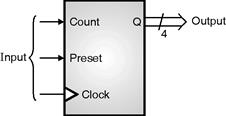Home > VHDL > Counters > Binary Down Counter

An n-bit binary counter can be constructed using a modified n-bit register where the data inputs for the register come from a decrementer (subtractor) for a down counter. Starting with a value stored in a register, to get to the next down count sequence, we simply have to substract a one to it.

A 4-bit binary down counter with asynchronous preset :

The behavioral VHDL code for the 4-bit binary down counter is shown in Fig. 2.13.2. The statement USE IEEE.STD_LOGIC_UNSIGNED.ALL is needed in order to perform subtractions on STD_LOGIC_VECTORs.library ieee;

use ieee.std_logic_1164.all;

use ieee.std_logic_unsigned.all; -- need this to subtract std_logic_vectors

entity counter is

port (clock: in std_logic;

preset: in std_logic;

count: in std_logic;

q : out std_logic_vector(3 downto 0));

end counter;

architecture behav of counter is

signal value: std_logic_vector(3 downto 0);

begin

process (clock, clear)

begin

if preset = '1' then

value <= (others => '1'); -- 4-bit vector of 1, same as "1111"

elsif (clock'event and clock='1') then

if count = '1' then

value <= value - 1;

end if;

end if;

end process;

q <= value;

end behav;